﻿ Kinematic Analysis

# DipAnalyst 2.0

## Kinematic Analysis

Kinematic analysis is a method used to analyze the potential for the various modes of rock slope failures (plane, wedge, toppling failures), that occur due to the presence of unfavorably oriented discontinuities (Figure 1). Discontinuities are geologic breaks such as joints, faults, bedding planes, foliation, and shear zones that can potentially serve as failure planes. Kinematic analysis is based on Markland’s test which is described in Hoek and Bray (1981). According to the Markland’s test, a plane failure is likely to occur when a discontinuity dips in the same direction (within 200) as the slope face, at an angle gentler than the slope angle but greater than the friction angle along the failure plane (Hoek and Bray, 1981) (Figure 1). A wedge failure may occur when the line of intersection of two discontinuities, forming the wedge-shaped block, plunges in the same direction as the slope face and the plunge angle is less than the slope angle but greater than the friction angle along the planes of failure (Hoek and Bray, 1981) (Figure 1). A toppling failure may result when a steeply dipping discontinuity is parallel to the slope face (within 300) and dips into it (Hoek and Bray, 1981). According to Goodman (1989), a toppling failure involves inter-layer slip movement. The requirement for the occurrence of a toppling failure according to Goodman (1989) is “If layers have an angle of friction Φj, slip will occur only if the direction of the applied compression makes an angle greater than the friction angle with the normal to the layers. Thus, a pre-condition for interlayer slip is that the normals be inclined less steeply than a line inclined Φj above the plane of the slope. If the dip of the layers is σ, then toppling failure with a slope inclined α degrees with the horizontal can occur if (90 - σ) + Φj < α”.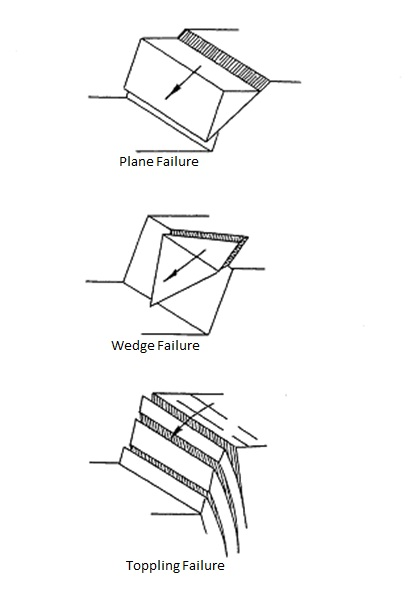Figure 1: Slope failures associated with unfavorable orientation of discontinuities (modified after Hoek and Bray, 1981).

Stereonets are used for graphical kinematic analysis. Stereonets are circular graphs used for plotting planes based on their orientations in terms of dip direction (direction of inclination of a plane) and dip (inclination of a plane from the horizontal). Orientations of discontinuities can be represented on a stereonet in the form of great circles, poles or dip vectors. Clusters of poles of discontinuity orientations on stereonets are identified by visual investigation or using density contours on stereonets (Hoek and Bray, 1981). Single representative orientation values for each cluster set is then assigned. These single representative orientation values, can be the highest density orientation value within a cluster set, or the mean dip direction/dip of a pole cluster as calculated using equations in Borradaille (2003).

Great circles for representative orientation values along with great circle for slope face and the friction circle are plotted on the same stereonet to evaluate the potential for discontinuity-orientation dependent failures (Figures 2,3,4) (Hoek and Bray, 1981). This stereonet-based analysis is qualitative in nature and requires the presence of tight data clusters for which a reasonably representative orientation value can be assigned. The chosen representative value may or may not be a good representation for a cluster set depending on the tightness of data within a cluster. Tight circular data is more uniform and has less variation making representative values more meaningful than for cases where data shows a wide scatter. Fisher’s K value is used to describe the tightness of a scatter (Fisher, 1953). It is calculated as follows:

K = M – 1/M-|rn|, where M is no. of data within a cluster, and |rn| is the magnitude of resultant vector for the cluster set (Fisher, 1953).

High K values indicate tightly clustered data, i.e. well-developed cluster set. If the cluster set is tight, the representative values are more reliable and so is the stereonet-based kinematic analysis. However, there are cases when a tight circular clustering of discontinuity orientations does not exist. A different quantitative approach that does not require tightly clustered discontinuity sets can be performed by DipAnalyst 2.0. DipAnalyst 2.0 can also be used for the stereonet-based method.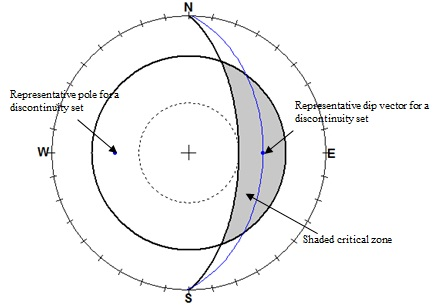Figure 2: Stereographic plot showing requirements for a plane failure (Hoek and Bray, 1981, Watts 2003). If the dip vector (middle point of the great circle) of the great circle representing a discontinuity set falls within the shaded area (area where the friction angle is higher than slope angle), the potential for a plane failure exists (figure created using RockPack).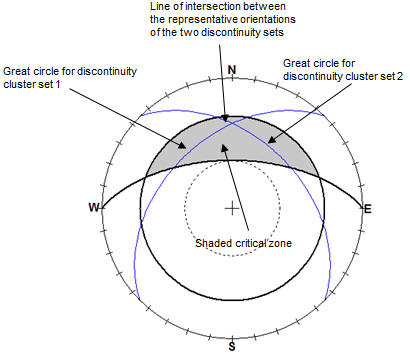Figure 3: Stereographic plot showing requirements for a wedge failure (Hoek and Bray, 1981, Watts 2003). If the intersection of two great circles representing discontinuities falls within the shaded area (area where the friction angle is higher than slope angle), the potential for a wedge failure exists (figure created using RockPack software).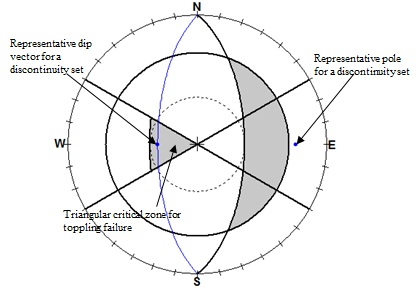Figure 4: Stereographic plot showing requirements for a toppling failure (Goodman, 1989, Watts 2003). The potential for a toppling failure exists if dip vector (middle point of the great circle) falls in the triangular shaded zone (figure created using RockPack software).

## Factor of Safety Calculations

Limit equilibrium analysis is used to calculate the factor of safety (F.S.) of a slope against failure if the kinematic analysis indicates the potential for failure. Factor of safety is the ratio of the resisting forces (shear strength) that tend to oppose the slope movement to the driving forces (shear stress) that tend to cause the movement along a plane of discontinuity. The equation for F.S. is:

F.S. = (c + s tan f)/t                                                                                                   (13)

Where: F.S. = factor of safety

c = cohesion

f = angle of internal friction

s = normal stress on slip surface

t = shear stress

According to the limit equilibrium approach, a factor of safety value equal to 1 represents limiting condition. A value greater than 1 represents a stable slope, and a value less than 1 indicates an unstable slope. The desired value of factor of safety depends upon the importance of the slope and the consequences of failure. For heavily travelled roads, slopes are usually designed to have a factor of safety equal to or greater than 1.3 under saturated conditions, maximum loads, and worst expected geological conditions (Canadian Geotechnical Society, 1992; Wyllie and Mah, 2004). The equations derived for both plane and wedge failure, consider weight of the sliding block, cohesion along plane of discontinuity, effect of water present along planes of discontinuity and tension applied from a rock bolt. Although the equations for factor of safety calculations of plane and wedge failures are based on Equation (13), they vary due to differences in the shape of the sliding block for the case of plane vs. wedge failures. The methods for calculating the factor of safety for plane and wedge failures with corresponding equations to determine the resisting and driving forces, including the effect of water pressure along discontinuities and application of a rock bolt are given in Hoek and Bray (1981) and Wyllie and Mah (2004).

To calculate factor of safety for plane failure, DipAnalyst 2.0, requires slope height, slope angle, discontinuity plane inclination, position and depth of tension crack, and ground water conditions along the discontinuity plane/tension crack (Figure 5).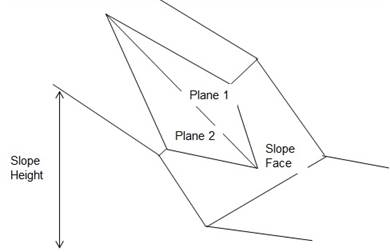Figure 5: Components of plane failure analysis

For wedge failure, DipAnalyst 2.0 follows the “short solution” put forward by Hoek and Bray (1981). The solution requires slope height, slope angle, orientation of the two intersecting planes (Figure 6). The “short solution” does not consider the effect of a rock bolt, and the presence of a tension crack.Figure 6: Components of wedge failure analysis.

REFERENCES

BORRADAILE, G., 2003, Statistics of Earth Science Data: Springer, New York, 351p.

FISHER, R.,A., 1953, Dispersion on a sphere: Proceedings of the Royal society of London, A217, pp.295-305.

GOODMAN, R. E., 1989, Introduction to Rock Mechanics: John Wiley & Sons, New York, NY 562 p.

HOEK, E. and BRAY, J. W., 1981, Rock Slope Engineering: The Institute of Mining and Metallurgy, London, England, 358 p.

WATTS,C.F., GILLIAM, D.R., HROVATIC,M.D., and HONGH, 2003, User’s Manual-ROCKPACK III for Windows: C.F.Watts and Associates, Radford, VA, 33 p.

WYLLIE, D. C. and MAH, C. W., 2004, Rock slope Engineering: 4th Edition, Spon Press, London and New York, 432p.